# Email Category Prediction

By accurately predicting the category of an email, we can better extract useful information from it according to its corresponding templates.

Introduction
Email category prediction can be seen as a multi-class classification task, since emails with similar themes may be automatically created using similar templates. The purpose of this paper is to predict a future email to be received by a user according to his previous emails. That is, if a user received a email with certain themes, several days later an email with the same or related templates may be sent to him.

The paper has very practical implications that can directly benefit our daily life. By accurately predicting the category of an email, we can better extract useful information from it according to its corresponding templates. This will be very helpful for email providers, for example, to automatically insert the user’s schedule into the his calendar, thus improving the user experience.

The paper uses MLP and LSTM neural networks, respectively, to make the prediction, in order to compare with the Markov Chain model previously used.

The MLP and LSTM models proposed by this paper have achieved some of the highest MRR scores, at 0.918 and 0.923 respectively, compared with 0.840 of the 5-dependent Markov Chain model, improving in prediction accuracy from state-of-the-art by around 9%.

Outside of the results, this paper highlights that in order to predict the category of a future email, not only is it important to consider the previously occurred categories, but also information about time-stamp, which is exactly what the paper chose to do when mapping the input features.

Three models in comparison

1. Markov Chain
One of the most influential models to solve the email category prediction problem was Markov Chain Model proposed by Gamzu et al. The Markov Chain can be expressed by ‘state pairs’ as:where a^(1) to a^(n) refers to a sequence of email categories for the n emails received by a user, sorted by received time-stamps in particular; and k refers to a prediction window we choose, which means that we consider k emails at each state, also called k-dependent Markov Chain. We can then build the directed graph, with the nodes as the different states, and the edge links between the two states in a state pair. The weight of each edge represents the ‘transition probability’ from one state to the other sate. This kind of Markov Chain model is used to predict the future email, and through combining with the temporal information, the model achieved a precision of about 45%.

2. Multilayer Perceptrons (MLP)
The first neural network model the paper proposes is the simple feedforward model: Multilayer Perceptron (MLP). In contrast to the ordinary sigmoid or hyperbolic tangent activation function, the paper uses rectiﬁed linear units (ReLUs) in its neurons, which better simulates the ‘sparse activation’ of real neurons, thus is more biologically plausible. (For example, for the widely used sigmoid function, nearly half of the neurons would be activated, which doe not match the actual situation of real neurons in our brain.) The calibration function is written as: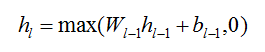Where h_l refers to the output of the l-th layer, W is the weights matrix between layer l-1 to layer l, and b is the bias vector.

If the MLP has L hidden layers, the output layer would first use a logit function to produce a vector z: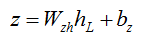Then, a probability vector is created using a softmax function as: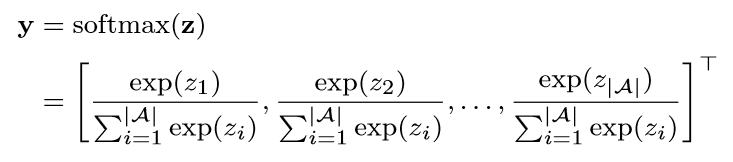Where A refers to the number of all email categories. The probability the future email belonging to the a-th category, P(a), is therefore [y]a .

The training loss function to be optimized is written as: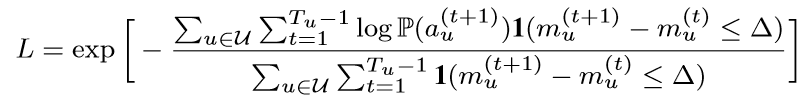Where u refers to a particular user, a_u^t refers to the category, and m_u^t the timestamp of the email received in time t. Delta is the size of the prediction time window, which means we consider emails that arrive in succession within Delta time. The 1 function has the value of 1 if the time gap between the two emails do not exceed Delta, and 0 vice versa (in the paper, Delta is set to be one week). Then, ‘Adam stochastic optimization algorithm’ is used to optimize L and ‘the truncated backpropagation through time algorithm’ is used to compute the gradient of L with respect to the parameter W and b (see D. Kingma and J. Ba. Adam,2014 and I. Goodfellow, Y. Bengio, and A. Courville,2016).

3. Long short-term memory
The second neural network model is Long short-term memory (LSTM), which turns out to be very efficient in solving sequential problems. Compared with the rather simple structure of MLP, LSTM has ‘memory cells’ in each layer that process the emails one by one, and store the output of the previous layer as well as the information of the previous email. A more formal expression of it can be written as:We can understand it more clearly by the graph below:Looking further into how the ‘LSTM Block’ works, we can get a clearer picture on why the memory cell c can actually ‘memorize’:

h_1^0 and c_1^0 are initialized both as zero-vectors and in each processing state, the LSTM Block updates the parameters shown in the graph above simultaneously as:where sigma σ is a sigmoid function. σ and tanh are both element-wise, and the symbol in the last two equations is also element-wise multiplication operator.

Several highlights in this paper

1. Input mapping
As mentioned above, the paper proposes that the probability of the category of a future email not only relate to the categories of the previous emails, but also the temporal information of these emails. So, when mapping the input k received emails into real number vectors, each email vector consists of three parts:

• the ‘category vector’ a of this email using one-hot representation. (the length of the vector A is the number of all the categories, and the a-th dimension is set to 1, while the other dimensions are set to 0). If we have a categories, the category vector will be a-dimensional.
• the ‘day-of-the-week vector’ d, also mapping by one-hot representation.(in the paper d is set to 7, for 7 days of a week).
• the ‘period-of-the-month vector’ p, again mapping by one-hot representation(in the paper p is set to 3, standing for the ‘beginning, middle and end’ of a month).

the time gap between two emails is set as real numbers. If it does not exceed one week, the value will be 1; and if it is longer than one week, the value will be 0. Therefore, the whole input vector of k emails will have k*s values, with s as the length of each email vector, and s=a+d+p+1.

2. Dataset Splitting
The paper uses 102,743 users and 2,562,361 emails received by them spanning 90 days, and these emails are divided into 17 categories.

The authors of the paper give mature consideration to all aspects of the question, and split the dataset in two ways as shown below. The first makes the test set contain those emails received by a known set of users, but unseen in training and validation set; while the second further ensures the emails in the test set belong to a new set of users.

3. Measuring the accuracy of the prediction
The paper uses Mean Reciprocal Rank (MRR) to measure the accuracy of the prediction. RR means the reciprocal of the position for the first correctly predicted category, sorted by decreasing order of the probability, also called rank; M means to compute the mean value of all the ranks.

Also, a success rate at r(SR@r) is used to measure the accuracy, which implies the proportion of the test emails whose ranks are smaller than or equals to r within the prediction time window.

For example, when splitting the dataset in the two ways mentioned above, and gradually increasing the prediction window k, the respective MRR and SR@r of a Markov Chain model are shown below.4. Overfitting prevention
The paper adopts the dropout regularization method for recurrent neural networks to reduce overfitting(see V. Pham, T. Bluche, C. Kermorvant, and J. Louradour, 2014). Briefly speaking, dropout means to randomly clear some neurons in the hidden layers (that is to say, set them to be zero). The figure below shows that with some positive dropout, the LSTM model can achieve higher MRR. The figure also shows that for a LSTM model, a single hidden layer is enough to achieve satisfactory results, while MLP may need more hidden layers to achieve the same results.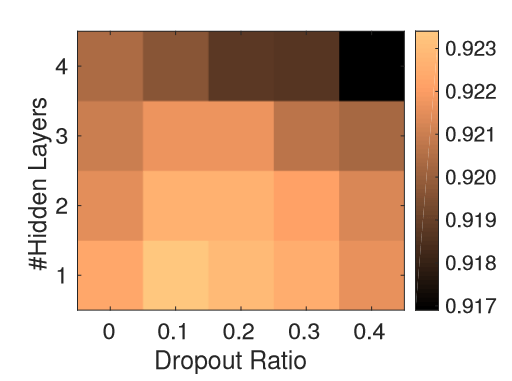Results
The experiment results provide insights in five aspects as follows:

a) The Markov chain model is not sufficient to describe the useful information of emails, because it cannot map the temporal features of the past emails very well. Because the MLP and LSTM models can better describe temporal information, they obviously outperform the Markov Chain model. The LSTM performs the best out of all three models.

b) Increasing the number of hidden layers above 2, and the number of neurons in each layer in both MLP and LSTM provides no significant improvement of the performance, as shown below:

c) Increasing the prediction window size k can greatly improve the accuracy only if k is no larger than 5, also shown in Figure 7. And increasing the prediction time window size improves accuracy too, also shown in Figure 6.

d) When using the second data splitting methods described in Figure 3, in which the users in the test set are unseen in the training and validation set, all three models achieve lower accuracy, but it is not very significant, despite the 5-dependent Markov Chain, as shown in the tables below.

e) if we minimize the ‘memory capabilities’ of the three models(for Markov Chain and MLP model, it means to set k=1, while for LSTM model, it means to clear all the memory cell), the performance of all the three models are slightly worse, also shown in the tables below.

Conclusion
The MLP and LSTM models proposed by this paper achieve the highest MRR scores of 0.918 and 0.923 respectively, compared to the 0.840 of the 5-dependent Markov Chain model. Even when clearing all the memory capabilities of the three models, the performance of LSTM is still the best.

This is a rather comprehensive experiment, and further improvement may be made on figuring out how to map more features of past emails, rather than the current category information and temporal information.

Comment from the reviewer
From the paper, we have seen that LSTM Neural Network achieved the best performance out of all three models. In fact, LSTM works pretty well in a broad range of problems, especially sequential problems (both time and space). One of the most impressive usages of LSTM is in Natural Language Processing, from POS tagging, syntactic structure analysis, to machine translation and speech recognition tasks, all of which requires the model to be able to process long-term context or dependencies between symbols in the input sequence.

Some languages that also have fixed ‘grammar templates’, such as Connectives in Chinese and Japanese, in which some connectives is very likely to appear after some other ‘pair’ connectives. The methods proposed in this paper may provide inspiration for improvements in Natural language Generation or Natural Language Understanding.

Reference
 Aston Zhang, Lluis Garcia-Pueyo, James B. Wendt, Marc Najork, Andrei Broder, Email Category Prediction
 D. Kingma and J. Ba. Adam: A method for stochastic optimization. arXiv preprint:1412.6980, 2014.
 V. Pham, T. Bluche, C. Kermorvant, and J. Louradour. Dropout improves recurrent neural networks for handwriting recognition. In 14th International Conference on Frontiers in Handwriting Recognition, pages 285–290, 2014.

Paper author: Aston Zhang, Lluis Garcia-Pueyo, James B. Wendt, Marc Najork, Andrei Broder University of Illinois at Urbana-Champaign, IL, USA, Google, CA, USA

Author: Kejin Jin | Reviewer: Hao Wang1.